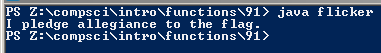# Assignment #91 and Flicker Phase

## Code

```    //Name: Mark Katz
///Period: 6
///Program Name: Flicker Phase
///File Name: flicker.java
///Date Finished: 11/13/15

import java.util.Random;

public class flicker
{
public static void main( String[] args )
{
Random rng = new Random();
int r;

for ( int i=0; i< 100000; i++ )
{
r = 1 + rng.nextInt(5);

if ( r == 1 )
{
first();
}
if ( r == 2 )
{
second();
}
if ( r == 3 )
{
third();
}
if ( r == 4 )
{
fourth();
}
if ( r == 5 )
{
fifth();
}

}

System.out.println("I pledge allegiance to the flag.");

}

public static void first()
{
System.out.print("I                               \r");
}

public static void second()
{
System.out.print("  pledge                        \r");
}

public static void third()
{
System.out.print("         allegiance             \r");
}

public static void fourth()
{
System.out.print("                    to the      \r");
}

public static void fifth()
{
System.out.print("                           flag.\r");
}
}

```

### Picture of the output